Games
Problems
Go Pro!

# Functions

Lesson Plans > Mathematics > Algebra > Functions

# Featured Lesson Plans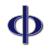Fibonacci Numbers and The Golden Ratio are the basis for many interesting problem solving questions and math classroom activities.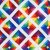An example of arithmetic series and geometric series, with application for math classes, or leadership conferences and seminars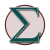Formulas and homework problems for sequences and series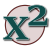Logarithm worksheet - basic problems for students learning the fundamental properties of logarithms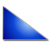Trigonometry and Anatomy have an etymological connection. This math lesson plan shows the strange word development of 'sine'.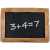Using a story about Karl Freidrich Gauss's childhood schooling to illustrate a point about arithmetic sequences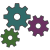Help students work through a challenging math problem involving interest compounded continuouslyA unit on basic right angle trigonometry as preparation for a Physics course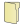# Full Directory Listing

Composite Functions and Function Values, Fibonacci Numbers and the Golden Ratio, Function Composition, Function Domain WorksheetLike us on Facebook to get updates about new resources